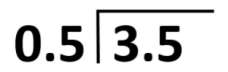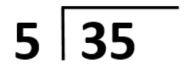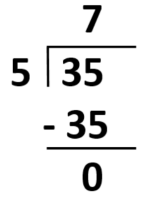# Decimals & Fractions

Decimals and fractions are important concepts in mathematics that allow us to represent and compare quantities that are not whole numbers. Understanding and being able to work with decimals and fractions is crucial for success in school and in many real-world situations, such as budgeting and shopping.

For example, if you want to buy a shirt that costs $19.99, you need to be able to understand that the decimal point separates the dollars from the cents and that the number to the right of the decimal point represents cents. If you don’t understand decimals, you might think that the shirt costs$1999, which is a very different price!

In addition to being useful in everyday life, decimals and fractions are often tested on standardized and selective admission tests, such as the ACT, SAT, and GRE. These tests often include questions that require you to convert between fractions and decimals, compare the size of fractions and decimals, and perform arithmetic operations with them.

To do well on these tests and succeed in math class, it’s important to practice working with decimals and fractions and to understand the concepts behind them. This includes understanding the place value of each digit in a decimal, the rules for multiplying and dividing decimals, and how to add, subtract, multiply, and divide fractions.

In summary, decimals and fractions are important concepts in math that are useful in everyday life and are frequently tested on standardized and selective admission tests. To succeed in these tests and in math class, it’s essential to practice and understand these concepts.

### Subtraction

Line up the decimal point of the given numbers, and subtract the values. Similar to addition, if a decimal point is not visible (i.e., whole numbers), the decimal is behind the number, and put zeroes after the decimal point.

### Multiplication

For example: 43.79 x 7 and 62.59 x 3.12 Step

Step 1: Multiply like the whole numbers.

Step 2: Count the number of decimal places in the factors.

Step 3: Place the decimal point in the product. The decimal place in the product is equal to the total number of decimal places in the factors.

### Division

For example: 3.5 ÷ 0.5

Step 1: Write out the long division form of the problem.Step 2: Transforming both decimal numbers to whole numbers will make the problem easier to solve. In the example, multiply both numbers with 10 to make them whole numbers.

Step 3: Solve the problem.Therefore, 3.5 ÷ 0.5is equals to 7.

## F R A C T I O N S

Like Fractions – directly add the numerators and retain the denominator.

Unlike Fractions

Unlike Fractions

Mixed Number

A Whole Number and a Fraction

### Subtraction

Like Fractions – directly subtract the numerators and retain the denominator.

Unlike Fractions

Unlike Fractions

### Division

Mixed Numbers
Step 1
: Convert the mixed numbers to an improper fraction.
Step 2: Just like dividing unlike fractions, keep the dividend and transform the divisor to its reciprocal (turning the fraction upside down).
Step 3: Multiply their numerators.
Step 4: Multiply their denominators.
Step 5: Simply the fraction.

A Whole Number and a Fraction
Step 1
: Convert the whole number into a fraction by taking the denominator as 1.
Step 2: Keep the dividend and transform the divisor to its reciprocal (turning the fraction upside down).
Step 3: Multiply their numerators.
Step 4: Multiply their denominators.
Step 5: Simply the fraction.